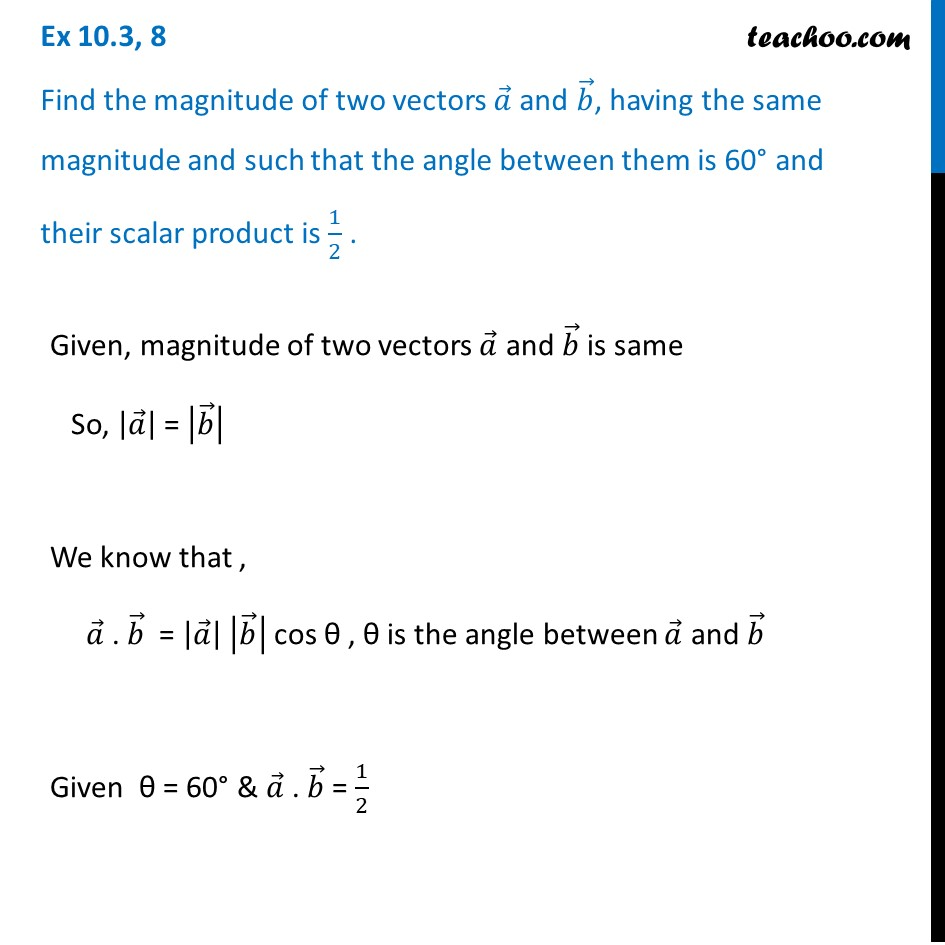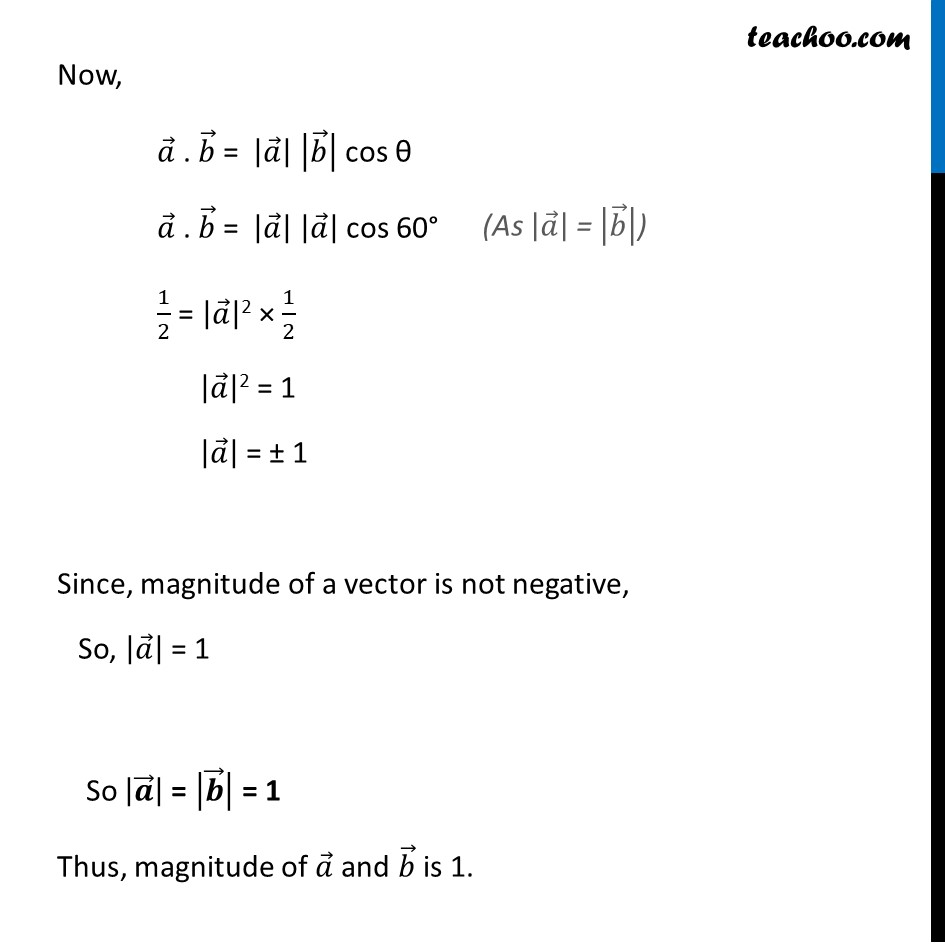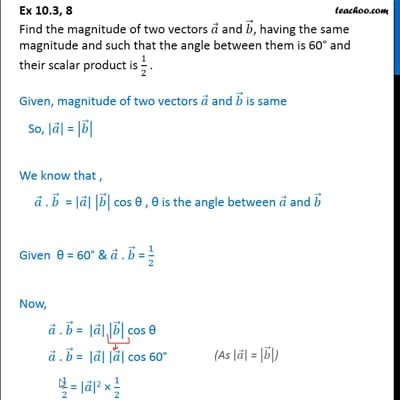Ex 10.3

Chapter 10 Class 12 Vector Algebra
Serial order wiseThis video is only available for Teachoo black users

Solve all your doubts with Teachoo Black (new monthly pack available now!)

### Transcript

Ex 10.3, 8 Find the magnitude of two vectors 𝑎 ⃗ and 𝑏 ⃗, having the same magnitude and such that the angle between them is 60° and their scalar product is 1/2 . Given, magnitude of two vectors 𝑎 ⃗ and 𝑏 ⃗ is same So, |𝑎 ⃗ | = |𝑏 ⃗ | We know that , 𝑎 ⃗ . 𝑏 ⃗ = |𝑎 ⃗ | |𝑏 ⃗ | cos θ , θ is the angle between 𝑎 ⃗ and 𝑏 ⃗ Given θ = 60° & 𝑎 ⃗ . 𝑏 ⃗ = 1/2 Now, 𝑎 ⃗ . 𝑏 ⃗ = |𝑎 ⃗ | |𝑏 ⃗ | cos θ 𝑎 ⃗ . 𝑏 ⃗ = |𝑎 ⃗ | |𝑎 ⃗ | cos 60° 1/2 = |𝑎 ⃗ |2 × 1/2 |𝑎 ⃗ |2 = 1 |𝑎 ⃗ | = ± 1 Since, magnitude of a vector is not negative, So, |𝑎 ⃗ | = 1 So |𝒂 ⃗ | = |𝒃 ⃗ | = 1 Thus, magnitude of 𝑎 ⃗ and 𝑏 ⃗ is 1.